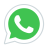# DC Circuit Analysis

Electrical Engineering is one of the major 4 year career course that a student takes at the university. Electrical engineering deals with the study of devices, inventions and products that use the con...
4 Students
Last updated 2022-02-13
Enrollment: 17
Language: English
\$3
\$23
This course has
Access on mobile
Certificate of Completion

• Electrical Engineering Basics
• Circuit Analysis Techniques
• Problem Solving Techniques
• Problem Analysis

1. Physics
2. Mathematics

## Which Students must take this course

1. Electrical Engineering Students
2. Computer Engineering Students
3. Mechatronics Engineering Students
4. Electronics Engineering Students
5. FSC students

## Course Content

Lecture 1: Introduction to Electrical and Electronics Engineering
00:07:28
Lecture 2: Circuit Analysis
00:15:53
Lecture 3: Circuit Analysis Techniques
00:04:26
Lecture 4: Ohm's Law
00:05:10
Lecture 5: Kirchhoff Current Law / KCL
00:21:21
Lecture 6: Kirchhoff Voltage Law / KVL
00:12:48
Lecture 7: Nodal Analysis
00:07:53
Lecture 8: Mesh Analysis
00:09:29
Lecture 9: Importance of Circuit Analysis
00:01:12

## Description

Electrical Engineering is one of the major 4 year career course that a student takes at the university. Electrical engineering deals with the study of devices, inventions and products that use the concepts of voltage and current. Circuits are the major part of the electrical engineering, computer engineering, mechatronics engineering, electronics engineering, telecommunication engineering. Hence it is important for an engineering student to understand the basics of Circuit Circuit Analysis is the field of analysing various circuit. This field also explain what are circuits and how they are build. How a circuit problem and what are some tools that help in solving the circuit problem. Circuit Analysis is importance because whatever the circuit you see in daily life i.e. computer, smartphone, medical equipment and etc uses the concept of voltage and current. This concept of voltage and current is well explained in circuit analysis Electrical Engineering DC Circuit Analysis is one of the major introduction courses on electrical engineering, electronics engineering, computer engineering and mechatronics engineering taught to undergraduate students

## Instructor Profile